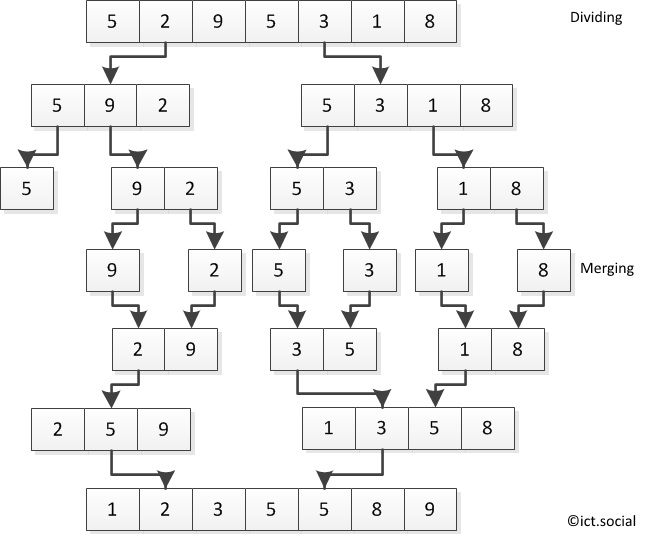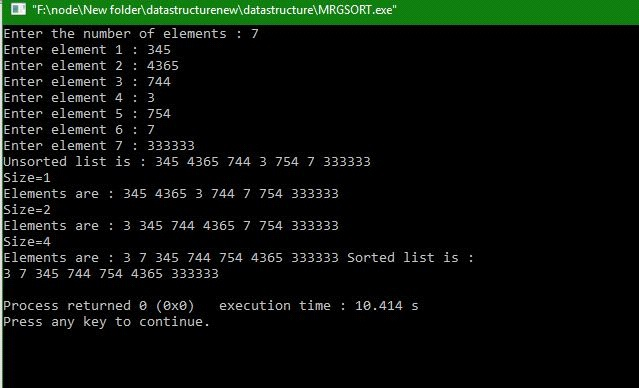# Merge Sort with and without Recursion using C program

In this article, we are going to learn about merge sort and implementing c program with and without using recursion.
Submitted by Manu Jemini, on January 24, 2018Image source: https://www.ict.social/images/19/algorithms/sorting/merge-sort.png

Merge Sort is base on divide and conquer algorithm.

1) DIVIDING

In Merge Sort, we take a middle index and break the array into two sub-arrays. These sub-array will go on breaking till the array have only one element.

2) MERGING

When all we have is single elements we start merging the elements in the same order in which we have divided them. During Merging, we also sort the sub-arrays, because sorting 10 arrays of 2 elements is cheaper than sorting an array of 20 elements.

In the end, we will have an array of elements, which is sorted.

## C program to implement Merge Sort using Recursion

```#include<stdio.h>

void merge(array, low, mid, high )
{
int temp[MAX];
int i = low;
int j = mid +1 ;
int k = low ;

while( (i <= mid) && (j <=high) )
{
if(array[i] <= array[j])
temp[k++] = array[i++] ;
else
temp[k++] = array[j++] ;
}/*End of while*/

while( i <= mid )
temp[k++]=array[i++];

while( j <= high )
temp[k++]=array[j++];

for(i= low; i <= high ; i++)
array[i]=temp[i];

}/*End of merge()*/

void merge_sort( int low, int high )
{
int mid;
if( low != high )
{
mid = (low+high)/2;
merge_sort( low , mid );
merge_sort( mid+1, high );
merge( low, mid, high );
}
}/*End of merge_sort*/

int main()
{
int i,n;

printf("Enter the number of elements : ");
scanf("%d",&n);
for(i=0;i<n;i++)
{
printf("Enter element %d : ",i+1);
scanf("%d",&array[i]);
}

printf("Unsorted list is :\n");
for( i = 0 ; i<n ; i++)
printf("%d ", array[i]);

merge_sort( 0, n-1);

printf("\nSorted list is :\n");
for( i = 0 ; i<n ; i++)
printf("%d ", array[i]);
printf("\n");

return 0;
}/*End of main()*/
```

## C program to implement Merge Sort without using Recursion

```#include <stdio.h>
#define MAX 30

int main()
{
int arr[MAX],temp[MAX],i,j,k,n,size,l1,h1,l2,h2;

printf("Enter the number of elements : ");
scanf("%d",&n);

for(i=0;i<n;i++)
{
printf("Enter element %d : ",i+1);
scanf("%d",&arr[i]);
}

printf("Unsorted list is : ");
for( i = 0 ; i<n ; i++)
printf("%d ", arr[i]);

/*l1 lower bound of first pair and so on*/
for(size=1; size < n; size=size*2 )
{
l1=0;
k=0;  /*Index for temp array*/
while( l1+size < n)
{
h1=l1+size-1;
l2=h1+1;
h2=l2+size-1;
/* h2 exceeds the limlt of arr */
if( h2>=n )
h2=n-1;

/*Merge the two pairs with lower limits l1 and l2*/
i=l1;
j=l2;

while(i<=h1 && j<=h2 )
{
if( arr[i] <= arr[j] )
temp[k++]=arr[i++];
else
temp[k++]=arr[j++];
}

while(i<=h1)
temp[k++]=arr[i++];
while(j<=h2)
temp[k++]=arr[j++];
/**Merging completed**/
/*Take the next two pairs for merging */
l1=h2+1;
}/*End of while*/

/*any pair left */
for(i=l1; k<n; i++)
temp[k++]=arr[i];

for(i=0;i<n;i++)
arr[i]=temp[i];

printf("\nSize=%d \nElements are : ",size);
for( i = 0 ; i<n ; i++)
printf("%d ", arr[i]);

}/*End of for loop */

printf("Sorted list is :\n");
for( i = 0 ; i<n ; i++)
printf("%d ", arr[i]);

printf("\n");

return 0;
}/*End of main()*/
```

Output